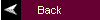MATLAB Tutorial3. Continuous Time System Analysis

Note, the recent versions of Matlab utilize a state space model to represent a system (where a system sys is defined as sys = ss(A,B,C,D)). Many of the commands that are listed below have sys as the preferred input argument rather than num and den. In many cases, the online help for Matlab does not even indicate the argument list as shown below; however, in most cases, the argument list as shown below still works. The authors purposely choose not to present the material in the book or in this tutorial using sys since it may obscure details for junior and sophomore-level students. For more details on this notation, see Section 3.F.

A. Transfer Function Representation

Commands covered:

tf2zp
zp2tf
cloop
feedback
parallel
series

Transfer functions are defined in MATLAB by storing the coefficients of the numerator and the denominator in vectors. Given a continuous-time transfer function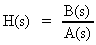where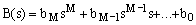andStore the coefficients of B(s) and A(s) in the vectors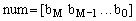and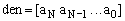. In this text, the names of the vectors are generally chosen to be num and den, but any other name could be used. For example,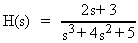is defined by

num = [2 3];
den = [1 4 0 5];

Note that all coefficients must be included in the vector, even zero coefficients.

A transfer function may also be defined in terms of its zeros, poles and gain: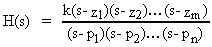To find the zeros, poles and gain of a transfer function from the vectors num and den which contain the coefficients of the numerator and denominator polynomials, type

[z,p,k] = tf2zp(num,den)

The zeros are stored in z, the poles are stored in p, and the gain is stored in k. To find the numerator and denominator polynomials from z, p, and k, type

[num,den] = zp2tf(z,p,k)

The overall transfer function of individual systems in parallel, series or feedback can be found using MATLAB. Store the transfer function G in numG and denG, and the transfer function H in numH and denH.

To reduce the general feedback system to a single transfer function, T(s) = G(s)/(1+G(s)H(s)) type

[numT,denT] = feedback(numG,denG,numH,denH);

For a unity feedback system, let numH = 1 and denH = 1 before applying the above algorithm. Alternately, use the command

[numT,denT] = cloop(numG,denG,-1);

To reduce the series system to a single transfer function, T(s) = G(s)H(s) type

[numT,denT] = series(numG,denG,numH,denH);

To reduce the parallel system to a single transfer function, T(s) = G(s) + H(s) type

[numT,denT] = parallel(numG,denG,numH,denH);

(Parallel is not available in the Student Version.)

back to list of contents

B. Time Simulations

Commands covered:

residue
step
impulse
lsim

The analytical method to find the time response of a system requires taking the inverse Laplace Transform of the output Y(s). MATLAB aides in this process by computing the partial fraction expansion of Y(s) using the command residue. Store the numerator and denominator coefficients of Y(s) in num and den, then type

[r,p,k] = residue(num,den)

The residues are stored in r, the corresponding poles are stored in p, and the gain is stored in k. Once the partial fraction expansion is known, an analytical expression for y(t) can be computed by hand.

A numerical method to find the response of a system to a particular input is available in MATLAB. First store the numerator and denominator of the transfer function in num and den, respectively. To plot the step response, type

step(num,den)

To plot the impulse response, type

impulse(num,den)

For the response to an arbitrary input, use the command lsim. Create a vector t which contains the time values in seconds at which you want MATLAB to calculate the response. Typically, this is done by entering

t = a:b:c;

where a is the starting time, b is the time step and c is the end time. For smooth plots, choose b so that there are at least 300 elements in t (increase as necessary). Define the input x as a function of time, for example, a ramp is defined as x = t. Then plot the response by typing

lsim(num,den,x,t);

To customize the commands, the time vector can be defined explicitly and the step response can be saved to a vector. Simulating the response for five to six time constants generally is sufficient to show the behavior of the system. For a stable system, a time constant is calculated as 1/Re(-p) where p is the pole that has the largest real part (i.e., is closest to the origin).

For example, consider a transfer function defined by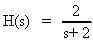The step response y is calculated and plotted from the following commands:

num = 2; den = [1 2];
t = 0:3/300:3; % for a time constant of 1/2
y = step(num,den,t);
plot(t,y)

For the impulse response, simply replace the word step with impulse. For the response to an arbitrary input stored in x, type

y = lsim(num,den,x,t);
plot(t,y)
back to list of contents

C. Frequency Response Plots

Commands covered:

freqs
bode
logspace
log10
semilogx
unwrap

To compute the frequency response H of a transfer function, store the numerator and denominator of the transfer function in the vectors num and den. Define a vector w that contains the frequencies for which H) is to be computed, for example w = a:b:c where a is the lowest frequency, c is the highest frequency and b is the increment in frequency. The command

H = freqs(num,den,w)

returns a complex vector H that contains the value of the frequency response for each frequency in w.

To draw a Bode plot of a transfer function which has been stored in the vectors num and den, type

bode(num,den)

To customize the plot, first define the vector w which contains the frequencies at which the Bode plot will be calculated. Since w should be defined on a log scale, the command logspace is used. For example, to make a Bode plot ranging in frequencies from 0.1 to 100, define w by

w = logspace(-1,2);

The magnitude and phase information for the Bode plot can then be found be executing:

[mag,phase] = bode(num,den,w);

To plot the magnitude in decibels, convert mag using the following command:

magdb = 20*log10(mag);

To plot the results on a semilog scale where the y-axis is linear and the x-axis is logarithmic, type

semilogx(w,magdb)

for the log-magnitude plot and type

semilogx(w,phase)

for the phase plot. The phase plot may contain jumps of ±2p which may not be desired. To remove these jumps, use the command unwrap prior to plotting the phase.

semilogx(w,unwrap(phase))

back to list of contents

D. Analog Filter Design

Commands covered:

buttap
cheb1ab
zp2tf
lp21p
lp2bp
lp2hp
lp2bs

MATLAB contains commands for various analog filter designs, including those for designing a Butterworth filter and a Type I Chebyshev filter. The commands buttap and cheb1ab are used to design lowpass Butterworth and Type I Chebyshev filters, respectively, with cutoff frequencies of 1 rad/sec. For an n-pole Butterworth filter, type

[z,p,k] = buttap(n)

where the zeros of the filter are stored in z, the poles are stored in p and the gain of the filter is in k. For an n-pole Type I Chebyshev filter with Rp decibels of ripple in the passband, type

[z,p,k] = cheb1ab(n,Rp)

To find the numerator and denominator polynomials of the resulting filter from z, p and k; type

[b,a] = zp2tf(z,p,k)

where a contains the denominator coefficients and b contains the numerator coefficients. Frequency transformations from one lowpass filter to another with a different cutoff frequency, or from lowpass to highpass, or lowpass to bandstop or lowpass to bandpass can be performed in MATLAB. These transformations can be used with either the Butterworth filters or the Chebyshev filters. Suppose b and a store the numerator and denominator of a transfer function of a lowpass filter with cutoff frequency 1 rad/sec. To map to a lowpass filter with cutoff frequency Wo and numerator and denominator coefficients stored in b1 and a1, type

[b1,a1] = lp2lp(b,a,Wo)

To map to a highpass filter with cutoff frequency Wo, type

[b1,a1] = lp2hp(b,a,Wo)

To map to a bandpass filter with bandwidth Bw centered at the frequency Wo, type

[b1,a1] = lp2bp(b,a,Wo,Bw)

To map to a bandstop filter with stopband bandwidth Bw centered about the frequency Wo, type

[b1,a1] = lp2bs(b,a,Wo,Bw)

back to list of contents

E. Control Design

Commands covered:

rlocus

Consider a feedback loop as shown in Figure 1 where G(s)H(s) = KP(s) and K is a gain and P(s) contains the poles and zeros of the controller and of the plant. The root locus is a plot of the roots of the closed loop transfer function as the gain is varied. Suppose that the numerator and denominator coefficients of P(s) are stored in the vectors num and den. Then the following command computes and plots the root locus:

rlocus(num,den)

To customize the plot for a specific range of K, say for K ranging from 0 to 100, then use the following commands:

K = 0:100;
r = rlocus(num,den,K);
plot(r,'.')

The graph contains dots at points in the complex plane that are closed loop poles for integer values of K ranging from 0 to 100. To get a finer grid of points, use a smaller increment when defining K, for example, K = 0:.5:100. The resulting matrix r contains the closed poles for all of the gains defined in the vector K. This is particularly useful to calculate the closed loop poles for one particular value of K. Note that if the root locus lies entirely on the real axis, then using plot(r,'.') gives inaccurate results.

back to list of contents

F. State Space Representation

Commands Covered:

ss
step
lsim
ss2tf
tf2ss
ss2ss

The standard state space representation is used in MATLAB, i.e.,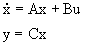where x is nx1 vector, u is mx1, y is px1, A is nxn, B is nxm, and C is pxn. The response of a system to various inputs can be found using the same commands that are used for transfer function representations: step, impulse, and lsim. The argument list contains the A, B, C, and D matrices instead of the numerator and denominator vectors. Alternately, the system can be combined into one model using the command

sys = ss(A,B,C,D);

Then, sys can be used as an input argument for the other commands. For example, the step response is obtained by typing either of the following commands:

[y,t,x] = step(A,B,C,D);
[y,t,x] = step(sys);

The states are stored in x, the outputs in y and the time vector, which is automatically generated, is stored in t. The rows of x and y contain the states and outputs for the time points in t. Each column of x represents a state. For example, to plot the second state versus time, type

plot(t,x(:,2))

To find the response of an arbitrary input or to find the response to initial conditions, use lsim. Define a time vector t and an input matrix u with the same number of rows as in t and the number of columns equaling the number of inputs. An optional argument is the initial condition vector x0. The command is then given as

[y,x] = lsim(A,B,C,D,u,t,x0) or [y,x] = lsim(sys,u,t,x0)

You can find the transfer function for a single-input/single-output (SISO) system using the command:

[num,den] = ss2tf(A,B,C,D);

The numerator coefficients are stored in num and the denominator coefficients are stored in den.

Given a transformation matrix P, the ss2ss function will perform the similarity transform. Store the state space model in A, B, C and D and the transformation matrix in P.

[Abar,Bbar,Cbar,Dbar]=ss2ss(A,B,C,D,P) or [Abar,Bbar,Cbar,Dbar] = ss2ss(sys,P)

performs the similarity transform z=Px resulting in a state space system that is defined as: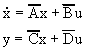where.

This tutorial is available as a supplement to the textbook Fundamentals of Signals and Systems Using Matlab by Edward Kamen and Bonnie Heck, published by Prentice Hall. The tutorial is designed for students using either the professional version of MATLAB (ver. 5.0) with the Control Systems Toolbox (ver. 4.0) and the Signal Processing Toolbox (ver. 4.0), or using the Student Edition of MATLAB (ver. 4.0). For more information on MATLAB, contact The Mathworks, Inc.